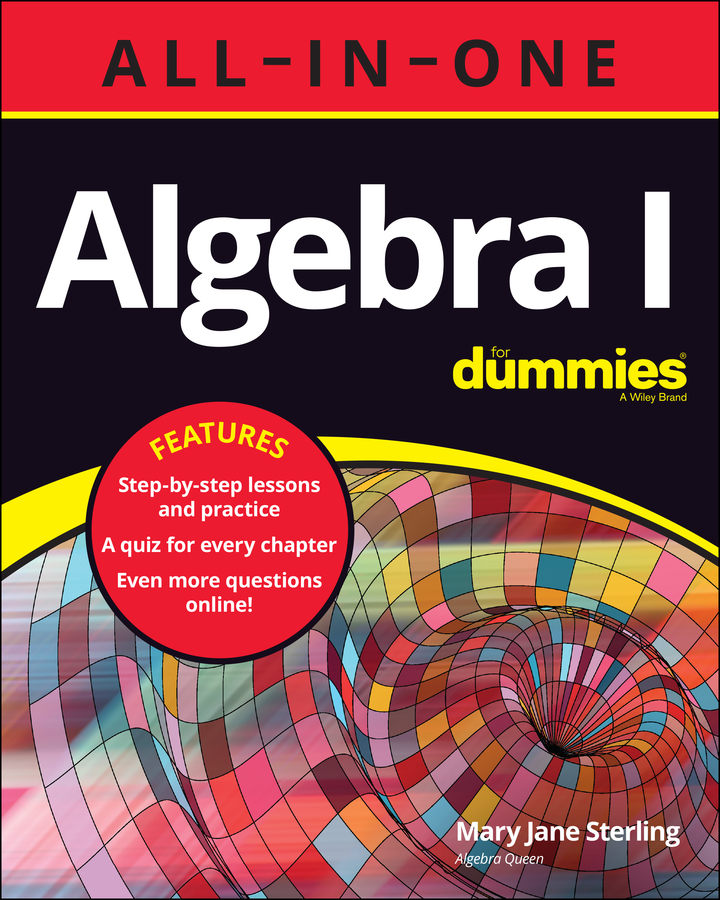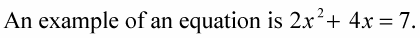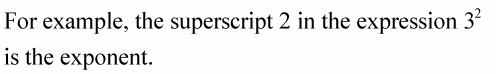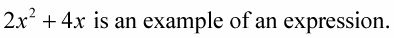##### Algebra I All-in-One For DummiesKnowing basic algebra vocabulary can help you translate key algebra words into algebra problems. By knowing the definitions of algebra vocabulary in this list, you will be able to construct and solve algebra problems much more easily.

• Constant. A value or number that never changes in an equation — it’s constantly the same.

For example, the number 5 is a constant because it is what it is; 5 is never 23 or some other number — 5 is always 5. A variable can be a constant if it is assigned a definite value. Usually, a variable representing a constant is one of the first letters in the alphabet.

For example, in the equation ax + bx + c + 0, a, b, and c are constants and the x is the variable. The value of x depends on what a, b, and c are assigned to be.

• Equation. A grouping of terms or values that use a sign to show an equal relationship — that two quantities are equal. By using an equation, tough problems can be reduced to easier problems and simpler answers.• ·Exponent. A small number written slightly above and to the right of a variable or number. It’s used to show repeated multiplication. An exponent is also called the power of the value.• Expression. Any combination of values and operations that can be used to show how things belong together and compare to one another.• Factor. To change two or more terms to just one term so that you can perform other processes. For example,

ab + ac +ad = a(b + c + d)

• Operation. An action performed upon one or two numbers to produce a resulting number. Operations are addition, subtraction, multiplication, division, square root, and so on.

For example, in the equation 3 + 4 = 7, the operation called for is addition.

• Simplify. To combine all that can be combined, reduce the number of terms, and put an expression in an easily understandable form.

One example is to simplify the fraction 2/4 to 1/2.

• Solve. To find the answer. In algebra, it means to figure out what the variable stands for.

For example, if you solve for x in the equation 4x = 20, you need to determine what number the variable x represents. So, divide 20 by 4, and you've solved the equation: x = 5!

• Term. A grouping together of one or more factors (variables and/or numbers), such as 4xy.

In this example, multiplication is connecting the number 4 with the variables x and y. Addition and subtraction, on the other hand, separate terms from one another.

For example, the expression 3xy + 5x − 6 has three terms.

• Variable. A letter that always represents an unknown number, or what you’re solving for in an algebra problem. A variable varies until it’s written in an equation or inequality, which is a comparison of two values. Then the fate of the variable is set — it can be solved for, and its value becomes the solution of the equation.

For example, x and y are variables, and the number that each represents isn't set until x and y become part of an equation, such as 2x + 4y = 20.